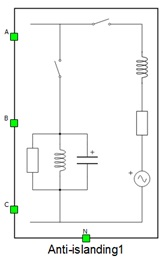# Anti-islanding Container

Description of the Anti-islanding component in Schematic Editor.

The Anti-islanding component is composed of three phase switches, three phase voltage source, passive components, and measurements. The internal structure of the anti-islanding container is shown in Figure 1. Phase voltage and phase current measurements are included, as shown in Figure 1. In Table 1, the Schematic Editor symbol and the Properties window of the grid simulator are shown.

Table 1. Anti-islanding component
component component dialog window component parametersAnti-islanding• Resistance of RLC load per phase (Ω)
• Inductance of RLC load per phase (H)
• Capacitance of RLC load per phase (F)
• Grid resistance per phase (Ω)
• Grid inductance per phase (H)

The list of available measurements of anti-islanding is shown in Internal analog measurements of the Anti-islanding component

## Internal analog measurements of the Anti-islanding component

Analog output variable name Description
(name).I_EUT_a Phase A current of device under test.
(name).I_EUT_b Phase B current of device under test.
(name).I_EUT_c Phase C current of device under test.
(name).I_grid_a Phase A grid current.
(name).I_grid_b Phase B grid current.
(name).I_grid_c Phase C grid current.
(name).V_grid_a Phase A grid voltage after grid impedance.
(name).V_grid_b Phase B grid voltage after grid impedance.
(name).V_grid_c Phase C grid voltage after grid impedance.
(name).Vgrid_A Phase A grid source voltage.
(name).Vgrid_B Phase B grid source voltage.
(name).Vgrid_C Phase C grid source voltage.
(name).inductance_phase_A Phase A grid inductance current.
(name).inductance_phase_B Phase B grid inductance current.
(name).inductance_phase_C Phase C grid inductance current.
(name).La Phase A RLC load inductance current.
(name).Lb Phase B RLC load inductance current.
(name).Lc Phase C RLC load inductance current.
(name).Ca Phase A RLC load capacitance voltage.
(name).Cb Phase B RLC load capacitance voltage.
(name).Cc Phase C RLC load capacitance voltage.